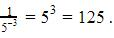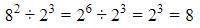Courses

# Test: Exponents And Powers- 2

## 40 Questions MCQ Test Mathematics (Maths) Class 8 | Test: Exponents And Powers- 2

Description
This mock test of Test: Exponents And Powers- 2 for Class 8 helps you for every Class 8 entrance exam. This contains 40 Multiple Choice Questions for Class 8 Test: Exponents And Powers- 2 (mcq) to study with solutions a complete question bank. The solved questions answers in this Test: Exponents And Powers- 2 quiz give you a good mix of easy questions and tough questions. Class 8 students definitely take this Test: Exponents And Powers- 2 exercise for a better result in the exam. You can find other Test: Exponents And Powers- 2 extra questions, long questions & short questions for Class 8 on EduRev as well by searching above.
QUESTION: 1

### The value of 25 is _________.

Solution: The value of 2 raised to power 5 is 32 because 2x2x2x2x2=32 therefore,32 is correct answer.
QUESTION: 2

### The Base in the expression 1024 is __________.

Solution: Answer is b not d. Base is 10 and exponent or power is 24.
QUESTION: 3

### The value of 30 is ________.

Solution:
QUESTION: 4

Multiplicative  inverse of 7-2 is _________

Solution:
QUESTION: 5

Fill in the blank  am × an = a ........ where m and n are natural numbers:-

Solution:
QUESTION: 6

The exponential form of 1/8 × (3)-3 is given by which of the following expression:

Solution:
QUESTION: 7

The value of 1/3-2 is equal to

Solution:
QUESTION: 8

In exponential form 149,600,000,000 m is given by :

Solution:
QUESTION: 9

In simplified form (3-1 + 4-1 + 5-1)0 is equals to

Solution:
QUESTION: 10

The value of (2/3)-2 is

Solution:
QUESTION: 11

In standard form 21600000 is written as

Solution:
QUESTION: 12

Usual form of the expression 3 X 10-5 is given by

Solution:

Since the power is negative, we move 5 place to the left including 3.So answer is 0.00003

QUESTION: 13

1 micron is equals to

Solution:
QUESTION: 14

The approximate distance of moon from the earth is 384,467,000 m and in exponential form. This distance can be written as

Solution:
QUESTION: 15

7 × 10-5 m is the standard form of which of the following

Solution:
QUESTION: 16

The standard form of 4050000 is given by

Solution:
QUESTION: 17

Which one of the following is the value of 115

Solution:
QUESTION: 18

1/125 is the multiplicative inverse of

Solution:So it’s the multiplicative inverse of 1/125

QUESTION: 19

16 is the multiplicative inverse of

Solution:
QUESTION: 20

Value of (30 + 20) × 50 is

Solution:
QUESTION: 21

The value of 72 is

Solution:
QUESTION: 22

The Base in the expression 8100 is

Solution:
QUESTION: 23

The value of 10000 is

Solution:
QUESTION: 24

Multiplicative inverse of 5-2 is

Solution:
QUESTION: 25

Value of (23)2 is given by ...........

Solution:
QUESTION: 26

The value of 82 ÷ 23 is given by........

Solution:QUESTION: 27

The value of 1/5-3 is equal to ............

Solution:
QUESTION: 28

In exponential form 140,000,000,000 Kg is given by ............

Solution:
QUESTION: 29

The expression, (5-1 + 7-1 + 3-1)0 is equals to

Solution:
QUESTION: 30

The value of (1/3)-2 is

Solution:
QUESTION: 31

In standard form 56700000 is written as ............

Solution:
QUESTION: 32

Usual form of the expression 9 X 10-5 is given by

Solution:
QUESTION: 33

The number 86,800,000,000,000,000,000,000,000 Kg  is equals to

Solution:

Any number written in the form m x 10n is a standard form if m is between 1 and 10, so we have 23  zeros after 868  and two decimal places  to make 868 less than 10. So we have 8.68 x 1025K

QUESTION: 34

Charge of an electron is 0.000,000,000,000,000,000,16 coulomb and in exponential form it can be written as

Solution:

Any number written in the form m x 10n is a standard form if m is between 1 and 10, so we have 18 zeros before 16 and after decimal and one decimal place after 1 to make 16 less than 10. So we have 1.6 x 10-19.

QUESTION: 35

13 × 10-7 Km is the standard form of which of the following

Solution:
QUESTION: 36

The standard form of 9030000000 is given by

Solution:
QUESTION: 37

Which one of the following is the value of 35

Solution:
QUESTION: 38

32 is the multiplicative inverse of

Solution: 3^2. Multiplicative inverse is the reciprocal, so, 1/3^-2.
1/3^-2 = 1/9{ -3*-3=9}
QUESTION: 39

64 is the multiplicative inverse of

Solution:
QUESTION: 40

The value of 20 × 30 × 40 is

Solution: